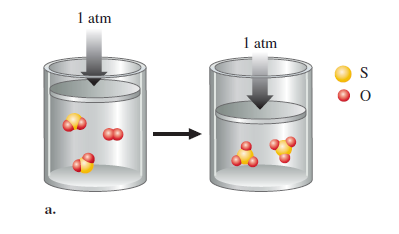# Problem: Three gas-phase reactions were run in a constant-pressure piston apparatus as shown in the following illustration. For each reaction, give the balanced reaction and predict the sign of w (the work done) for the reaction.If just the balanced reactions were given, how could you predict the sign of w for a reaction?

###### FREE Expert Solution
87% (437 ratings)
###### Problem Details

Three gas-phase reactions were run in a constant-pressure piston apparatus as shown in the following illustration. For each reaction, give the balanced reaction and predict the sign of w (the work done) for the reaction.If just the balanced reactions were given, how could you predict the sign of w for a reaction?

Frequently Asked Questions

What scientific concept do you need to know in order to solve this problem?

Our tutors have indicated that to solve this problem you will need to apply the First Law of Thermodynamics concept. You can view video lessons to learn First Law of Thermodynamics. Or if you need more First Law of Thermodynamics practice, you can also practice First Law of Thermodynamics practice problems.

What textbook is this problem found in?

Our data indicates that this problem or a close variation was asked in Chemistry: An Atoms First Approach - Zumdahl Atoms 1st 2nd Edition. You can also practice Chemistry: An Atoms First Approach - Zumdahl Atoms 1st 2nd Edition practice problems.